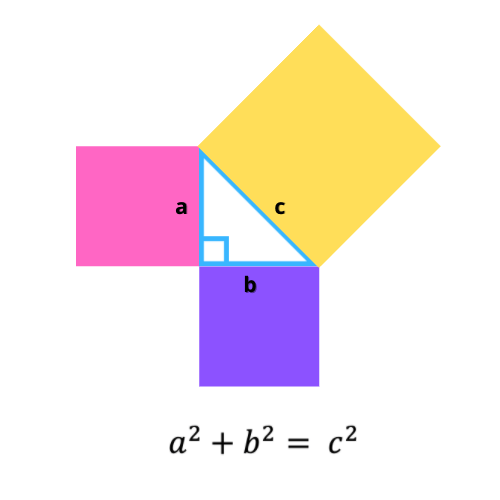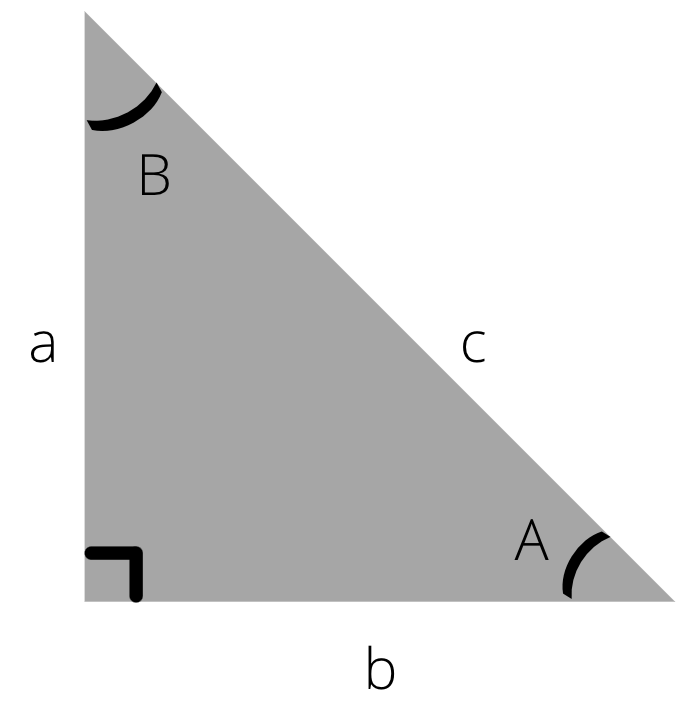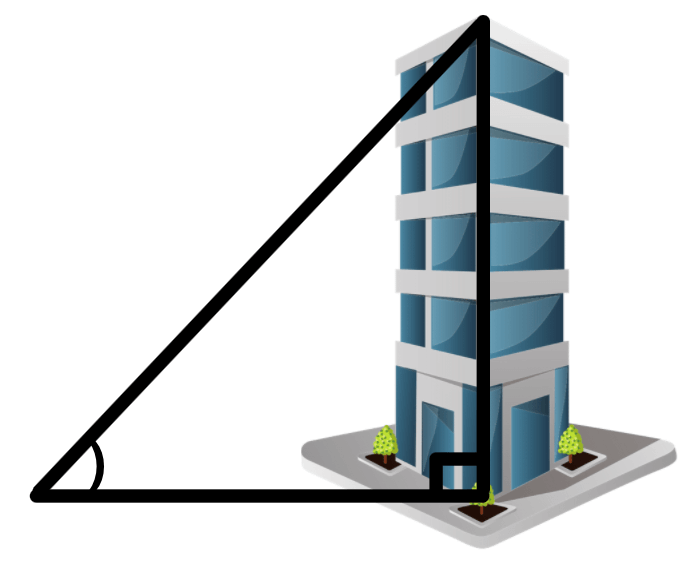Mathematical Calculators

# Right Triangle Side And Angle Calculator

FInd out easily right side and angle of an triangle with our free online calculator!

#### Calculate results with one side and one angle

 ◦What is a right triangle? ◦Pythagorean theorem ◦Formulas of the right triangle ◦Daily life examples of the right triangle

## What is a right triangle?

A right triangle (American English) is a triangle that has one right angle (90°). It is also known as a right-angled triangle (British English), or more formally, an orthogonal triangle.Example of a right triangle

## Pythagorean theorem

the Pythagorean theorem, also known as Pythagora's theorem, relates the three sides of a right triangle. According to this formula, the area of the square of a square whose side is the hypotenuse of a triangle is equal to the sum of the areas on the two other sides.
See the visual demonstration below:Pythagorean theorem
Pythagorean theorem - Wikipedia

## Formulas of the right triangle

The right triangle has many useful formulas to utilize. You can use any of the formulas below to calculate the angles, sides, area, or perimeter of the right triangle. We will reference the triangle below for the following formulas:a^2+ b^2=c^2

sin A = a / c
cos A = b / c
tan A = a / b
sin B = b / c
cos B = a / c
tan B = b / a

### The area of a triangle

Area = a \* b / 2

### The perimeter of a triangle

Perimeter = a + b + c
Also, note that you will need the table below when making use of the trigonometric functions:For example, if you are using the tan B formula and calculating its value to be 1, then by looking at the table above, you will know that the value of the angle in question is to be 45°.

## Daily life examples of the right triangle

The right triangle has many relevant and valuable formulas that are used in mathematics and real life. Below you will see three of the most important uses of the right triangle:

### 1) Architecture and engineering

It is not too far off to think about the usage of the right triangle in architecture. It is mainly used to calculate the length of the diagonal connection that connects two lines. This is used to calculate the diagonal length of a roof's slope when designing a sloped roof. You would only need to know the height and length of the roof, and you're good to go!### 2) Electronics and electrical engineering

The right triangle is used for resolving math problems in electronics and electrical engineering, primarily when designing a model. Another example of the importance is when making aesthetic additions and making sure they don't disturb the function of the model.
However, the right triangle comes in very handy when working with circuits. See the below visual example for further demonstration and to understand how the right triangle logic translates into circuit logic.### 3) Land surveying (civil engineering)

Surveying has been a profession that has been around for a long time, at least as long as recorded history shows. This is done by a surveyor who has the job of accurately measuring the earth's surfaces on a large scale. You might have guessed the use of the right triangle by now; basically, it comes in when the surveyor needs to calculate the length, areas, and relative angles between the objects on the landscape.
The example below is an excellent visual demonstrator of what has been previously explained. A surveyor uses relevant formulas to calculate his/her distance from the mountain top or from anywhere else they pick.See the article below for further information on how surveying works:
Surveying - WikipediaArticle author
Parmis Kazemi
Parmis is a content creator who has a passion for writing and creating new things. She is also highly interested in tech and enjoys learning new things.

###### Right Triangle Side And Angle Calculator English
Published: Tue Nov 02 2021
In category Mathematical calculators